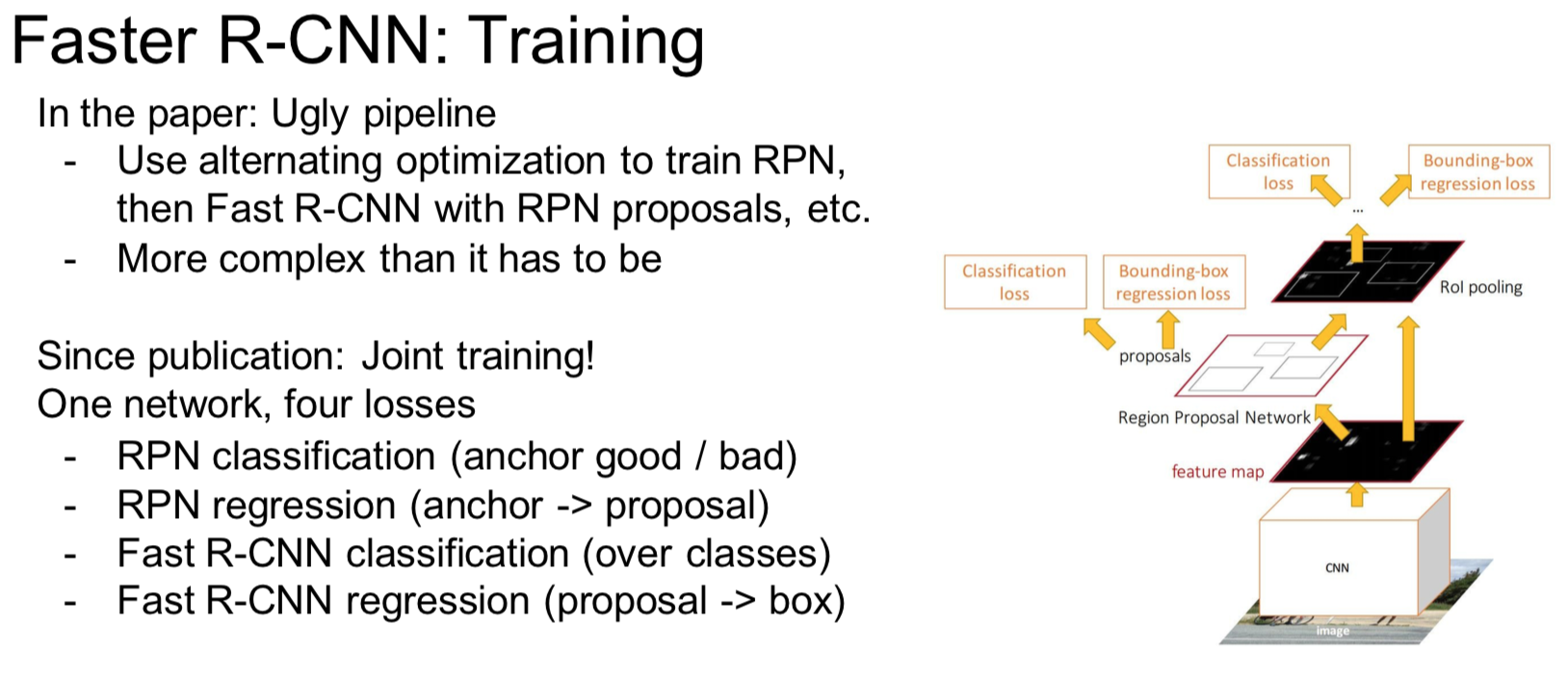# Faster R-CNN文章详细解读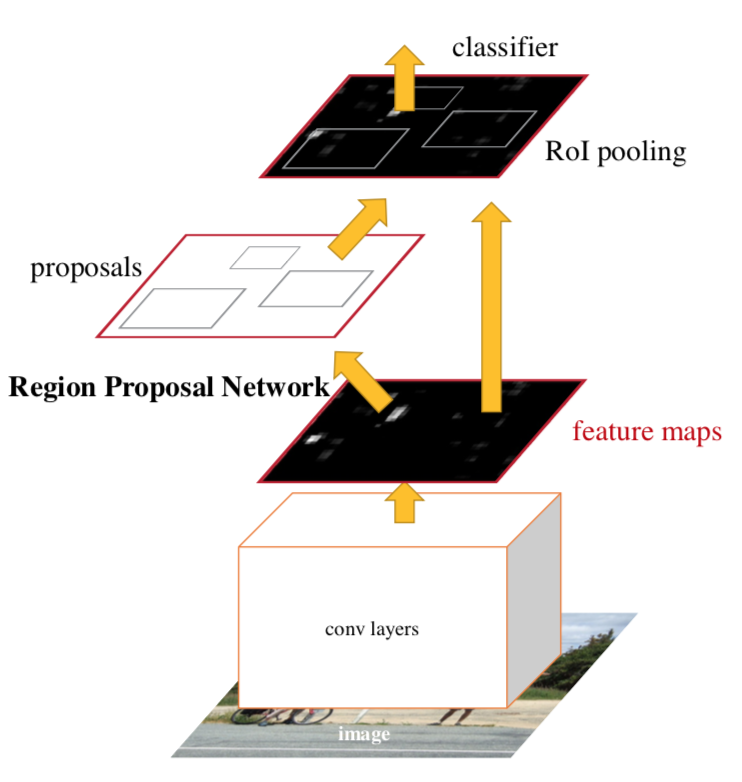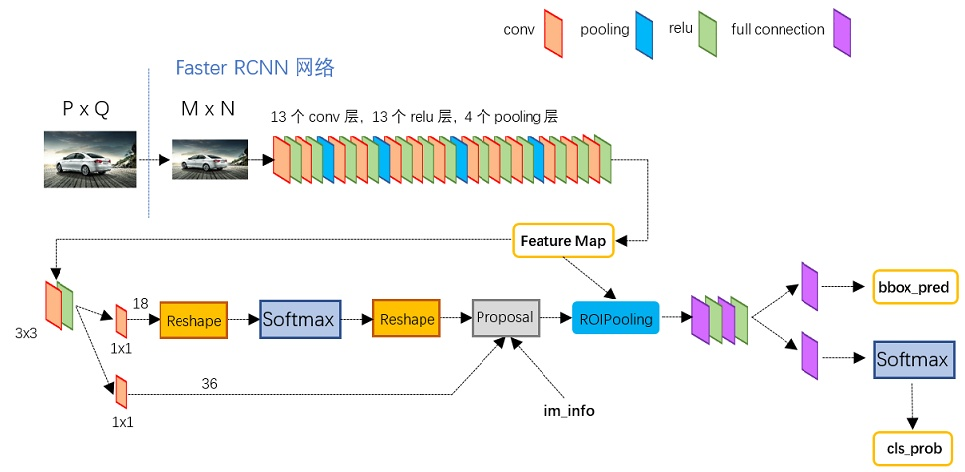1）卷积层(conv layers)，用于提取图片的特征，输入为整张图片，输出为提取出的特征称为feature maps
2）RPN网络(Region Proposal Network)，用于推荐候选区域，这个网络是用来代替之前的search selective的。输入为图片(因为这里RPN网络和Fast R-CNN共用同一个CNN，所以这里输入也可以认为是featrue maps)，输出为多个候选区域，这里的细节会在后面详细介绍。
3）RoI pooling，和Fast R-CNN一样，将不同大小的输入转换为固定长度的输出，输入输出和Faste R-CNN中RoI pooling一样。
4）分类和回归，这一层的输出是最终目的，输出候选区域所属的类，和候选区域在图像中的精确位置。

1.RPN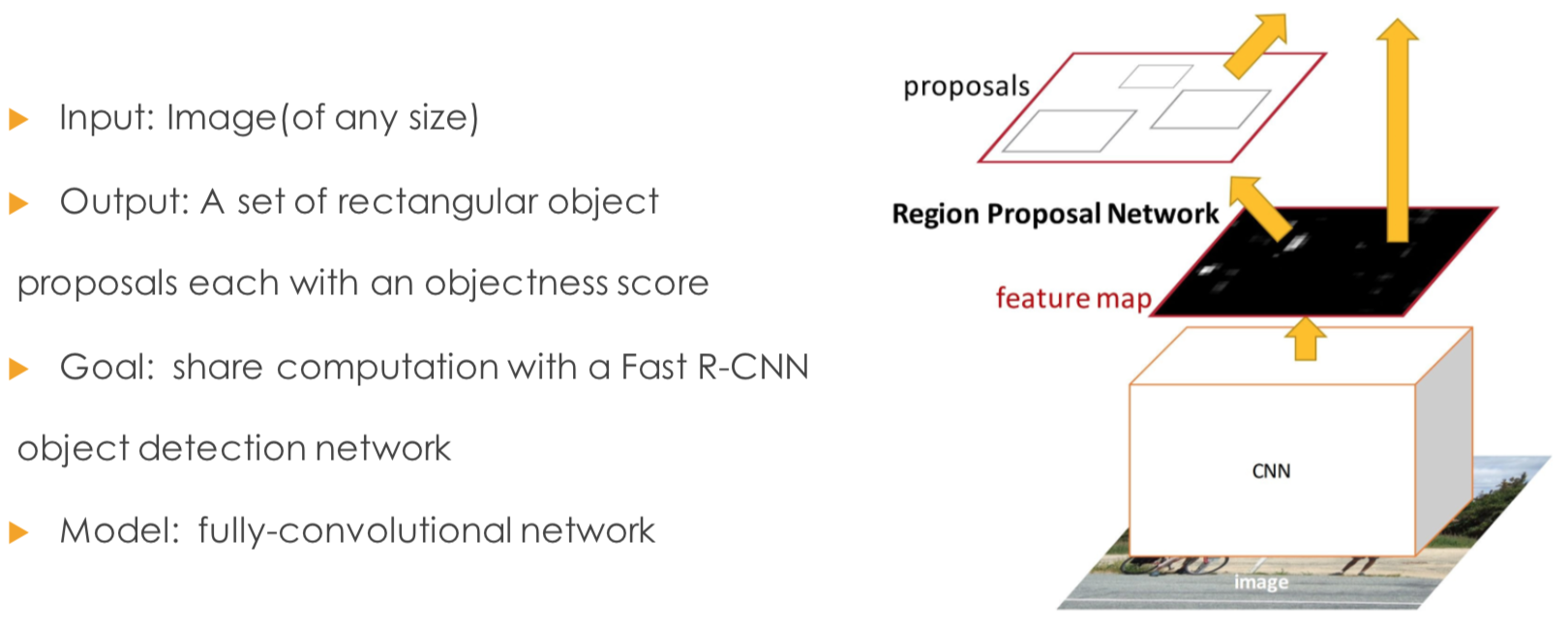2.anchors
anchors可以理解为一些预设大小的框，anchors的种类用k表示，在原文中k=9，由3种面积(${128}^{2}$$128^2$,${256}^{2}$$256^2$,${512}^{2}$$512^2$)和3种长宽比(1:1,1:2,2:1)组成，这里anchors的大小选取是根据检测时的图像定义，在检测时会将最小边缩放到600，最大边不超过1000（我看的是tf版本的代码）。生成anchors如下

[[ -84.  -40.   99.   55.]
[-176.  -88.  191.  103.]
[-360. -184.  375.  199.]
[ -56.  -56.   71.   71.]
[-120. -120.  135.  135.]
[-248. -248.  263.  263.]
[ -36.  -80.   51.   95.]
[ -80. -168.   95.  183.]
[-168. -344.  183.  359.]]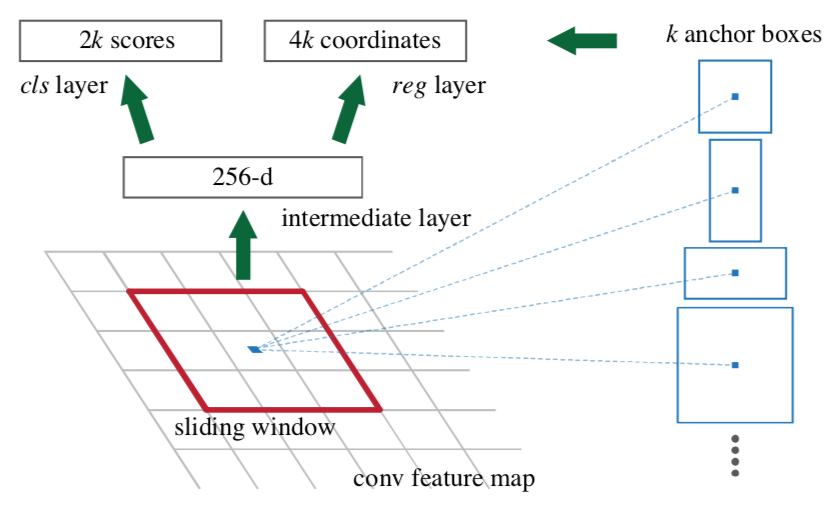1）在原文中使用的是ZF model中，其Conv Layers中最后的conv5层num_output=256，对应生成256张特征图(feature maps)，所以相当于feature map每个点都是256-dimensions
2）在conv5之后，做了rpn_conv/3x3卷积且num_output=256，相当于每个点又融合了周围3x3的空间信息），同时256-d不变
3）假设在conv5 feature map中每个点上有k个anchor（原文如上k=9），而每个anhcor要分foreground和background，所以每个点由256d feature转化为cls=2k scores；而每个anchor都有[x, y, w, h]对应4个偏移量，所以reg=4k coordinates（scores和coordinates为RPN的最终输出）
4）补充一点，全部anchors拿去训练太多了，训练程序会在合适的anchors中随机选取128个postive anchors+128个negative anchors进行训练(至于什么是合适的anchors接下来RPN的训练会讲)

3.RPN训练
RPN训练中对于正样本文章中给出两种定义。第一，与ground truth box有最大的IoU的anchors作为正样本；第二，与ground truth box的IoU大于0.7的作为正样本。文中采取的是第一种方式。文中定义的负样本为与ground truth box的IoU小于0.3的样本。

$L\left(\left\{{p}_{i}\right\},\left\{{t}_{i}\right\}\right)=\frac{1}{{N}_{cls}}\sum _{i}{L}_{cls}\left({p}_{i},{p}_{i}^{\ast }\right)+\lambda \frac{1}{{N}_{reg}}\sum _{i}{p}_{i}^{\ast }{L}_{reg}\left({t}_{i},{t}_{i}^{\ast }\right)$$L(\{p_{i}\},\{t_{i}\})=\frac{1}{N_{cls}}\sum_{i}L_{cls}(p_{i},p^{*}_{i})+\lambda\frac{1}{N_{reg}}\sum_{i}p^{*}_{i}L_{reg}(t_{i}, t^{*}_{i})$

4.RPN网络与Fast R-CNN网络的权值共享
RPN最终目的是得到候选区域，但在目标检测的最终目的是为了得到最终的物体的位置和相应的概率，这部分功能由Fast R-CNN做的。因为RPN和Fast R-CNN都会要求利用CNN网络提取特征，所以文章的做法是使RPN和Fast R-CNN共享同一个CNN部分。
Faster R-CNN的训练方法主要分为两个，目的都是使得RPN和Fast R-CNN共享CNN部分，如下图所示